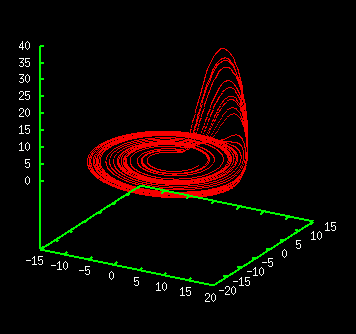# Rössler AttractorRösslor attractor is generated by three-dimensional differential equations written as

 dx/dt= -y -z dy/dt= x +ay dz/dt= b -cz +xz

The parameters are set as a=0.15, b=0.20, and c=10.0. Like Lorenz attractor, the dimension of the equation is three. Generally, to observe chaos in continuous time system, it is known that the dimension of the equation must be larger than three.

On the other hand, in discrete-time system like logistic map or Hénon map, we can see chaos even if the dimension is one.

You can observe the attractor from various view angles with "Rössler attractor" simulator.

<< Lorenz Attractor / Attractor of Hénon Map >>

Introduction to Chaos and Nonlinear Dynamics